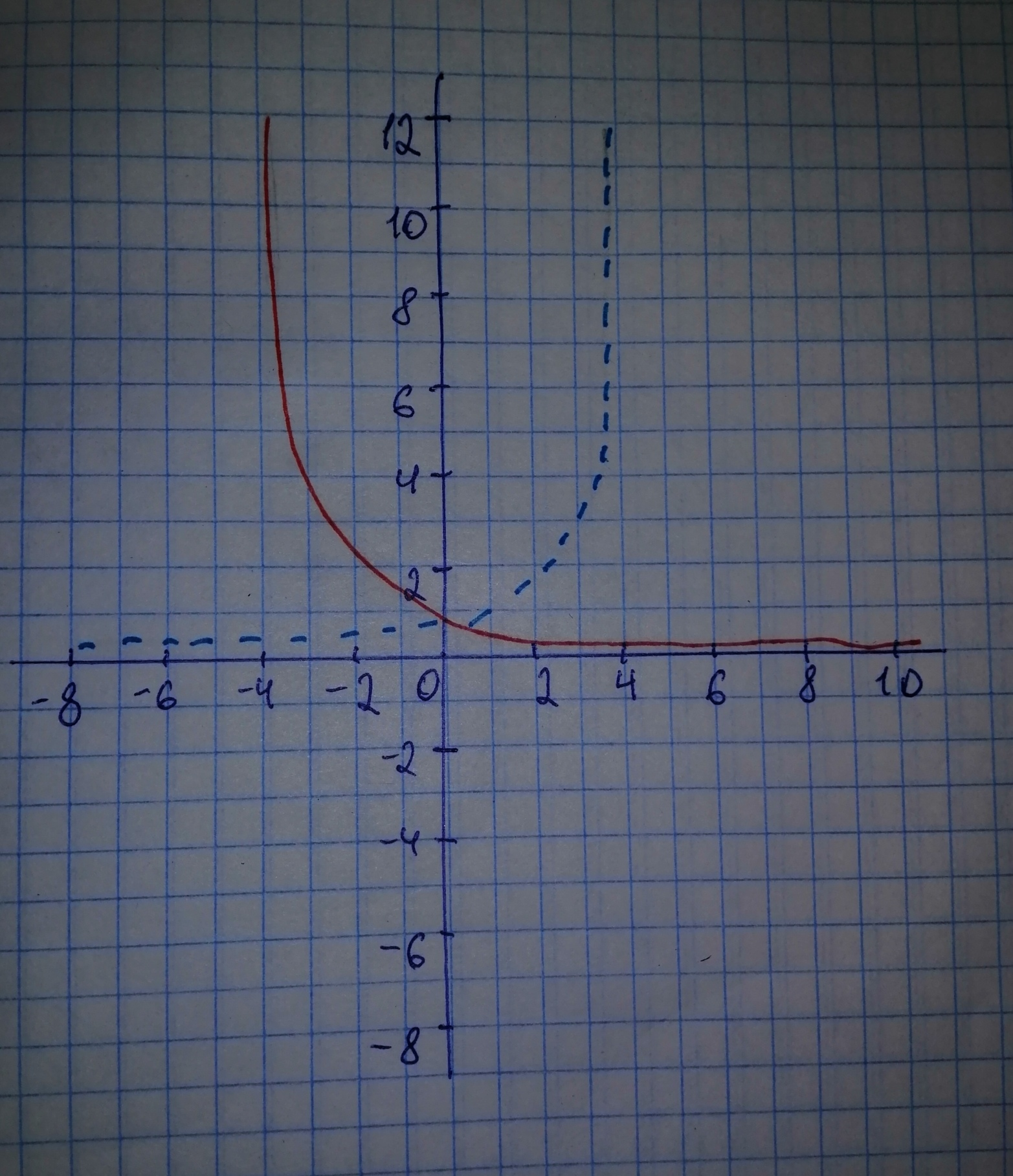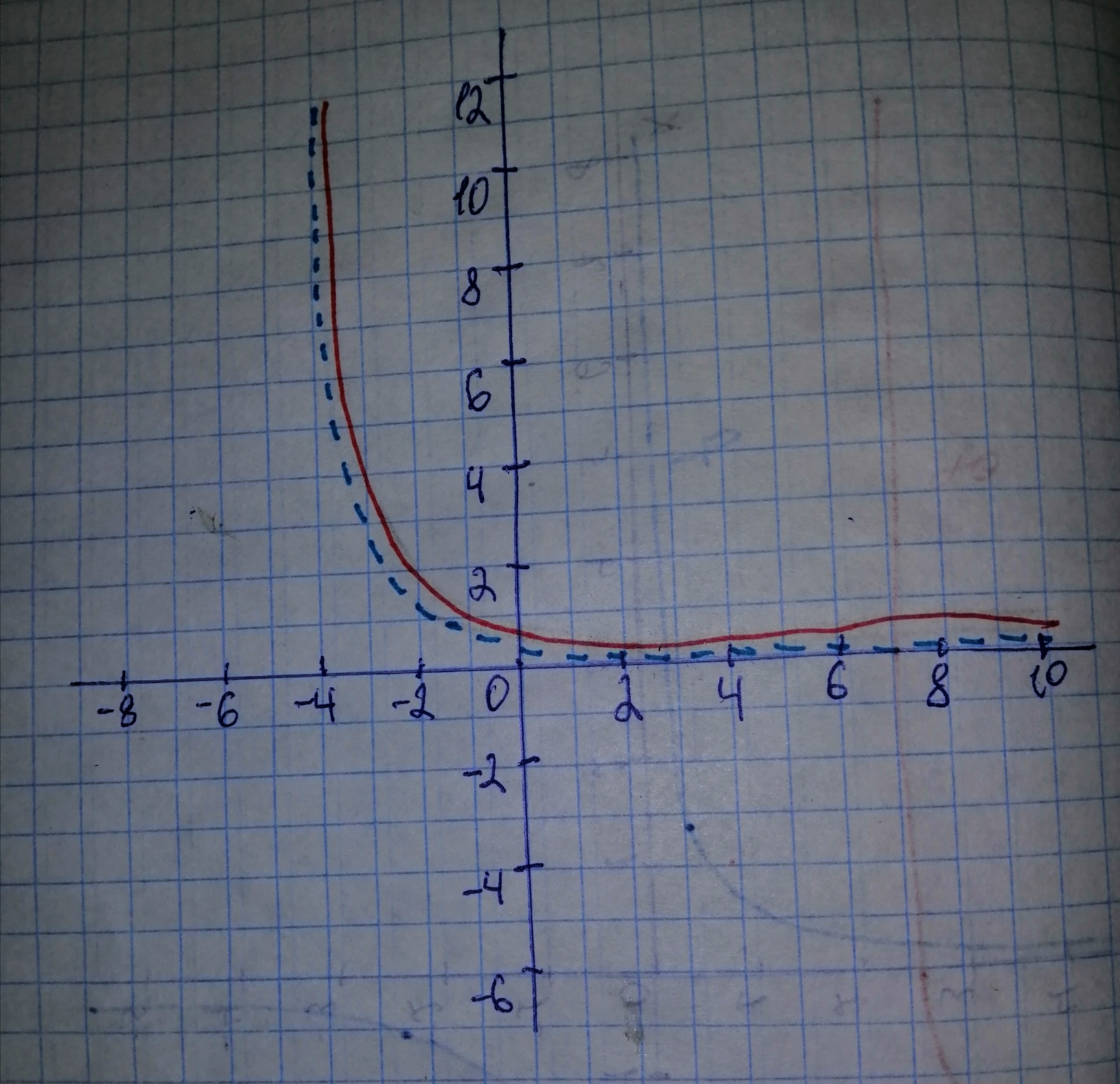Question# Use transformations to sketch the graph of the function. s(x)=1 + 0.5^{x}

Transformations of functions
ANSWEREDUse transformations to sketch the graph of the function. $$\displaystyle{s}{\left({x}\right)}={1}\ +\ {0.5}^{{{x}}}$$2020-11-07

Step 1
$$\displaystyle{s}{\left({x}\right)}={1}\ +\ {0.5}^{{{x}}}={1}\ +\ {\left(\frac{{1}}{{2}}\right)}^{{{X}}}={1}\ +\ {2}^{{-{x}}}$$
Remember that: When we reflect the graph of $$\displaystyle{y}={f{{\left({x}\right)}}}$$ in the y-axis, we get the graph for $$\displaystyle{y}={f{{\left(-{x}\right)}}}$$
We will start the graph of the standard function $$\displaystyle{y}={2}^{{{x}}}$$ (represented by blue dashed curve)
Reflect the graph of $$\displaystyle{y}={2}^{{{x}}}$$ in the y-axis, to get the graph for $$\displaystyle{y}={2}^{{-{x}}}$$ (represented by black solid curve)Step 2
Remember that: When we shift the graph of $$\displaystyle{y}={f{{\left({x}\right)}}}$$ by k units upwards, we get the graph for $$\displaystyle{y}={f{{\left({x}\right)}}}\ +\ {k}$$
Shift the graph of $$\displaystyle{y}={2}^{{-{x}}}$$ by 1 unit upwards, to get the graph for $$\displaystyle{y}={1}\ +\ {2}^{{-{x}}}$$ (represented by red solid curve).
The graph for $$\displaystyle{y}={2}^{{-{x}}}$$ is represented by black dashed curve.